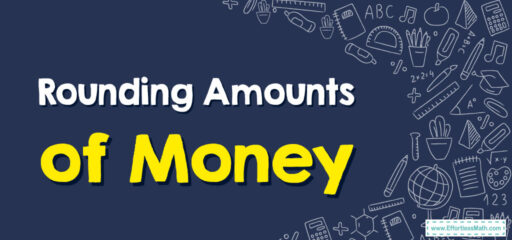# How to Round Amounts of Money

Rounding means making a number simpler but keeping its value close to what it was. With money, we often round to the nearest dollar or to the nearest ten cents.## A Step-by-step Guide to Rounding Amounts of Money

Here is a step-by-step guide to rounding amounts of money:

Money in dollars and cents has a decimal point. For example, $2.75 means 2 dollars and 75 cents. The first digit after the decimal point represents tens of cents (from 10 cents up to 90 cents), and the second digit represents single cents (from 1 to 9 cents). ### Step 2: Identify the Place Value You Are Rounding To Are you rounding to the nearest dollar, or to the nearest ten cents? If you’re rounding to the nearest dollar, you’ll be looking at the digit in the tenths place (the first digit after the decimal point). If you’re rounding to the nearest ten cents, you’ll be looking at the digit in the hundredths place (the second digit after the decimal point). ### Step 3: Apply the Rounding Rule If the digit you’re looking at is 5 or more, you round up, which means you add 1 to the number in the place you’re rounding to. If the digit is 4 or less, you round down, which means the number in the place you’re rounding to stays the same. ## Related to This Article ### More math articles ### What people say about "How to Round Amounts of Money - Effortless Math: We Help Students Learn to LOVE Mathematics"? No one replied yet. X 30% OFF Limited time only! Save Over 30% SAVE$5

It was $16.99 now it is$11.99